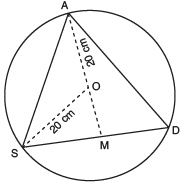Value-Based Questions: Circles

# Value-Based Questions: Circles - Notes | Study Mathematics (Maths) Class 9 - Class 9

 1 Crore+ students have signed up on EduRev. Have you?

Question 1. Amit, Deepak and Prabha have saved a good amount from their pocket money. They wish to donate it for a good cause. They sat on a round table of 20 cm radius, to decide the mode of donation. In the adjoining figure, Amit, Deepak and Prabha are sitting at A, S and D respectively, such that AS = SD = AD i.e., ∆ASD is an equilateral triangle.
(a) Find the distance between Amit, Deepak and Prabha.
(b) Which mathematical concept is used in the above problem?
(c) By donating the savings from the pocket money what values are depicted by Amit, Deepak and Prabha?Sol. (a) Since, AS = SD = AD [Given]
â ∆ASD is an equilateral triangle.
⇒ Perpendicular AM will pass through O.
â SM = (1/2) (SD) = x cm (say)                        [∵ Perpendicular from the centre to a chord, bisects the chord]
Now, in right angled ∆ASM, we have AM2 + SM=AS2
⇒ AM2 =AS2 – SM2
= (2 SM)2 – SM2                                    [∵ AS = SD and SM = (1/2) SD]
= 4SM2 – SM2 = 3 SM2 = 3x2
⇒ AM = √3x
Now, OM = AM – OA =                [ √3x – 20] cm
Also, in rt. ∆OBM, we have OS2 =SM2 + OM2
202 = x2 + OM2
⇒ 400 = x2 + ( √3x – 20)
⇒ 400 = x2 + 3x2 + 400 – 40 3x
⇒ 4x– 40 3x =0
⇒ 4x  (x – 10 √3= 0
⇒ x = 0 or x = 10√3
∵ x = 0 is not possible,
â x =10 3 cm
Now, SD = 2x cm = 2 × 10√3 cm = 20 √3 cm
⇒ Distance between Amit, Deepak and Prabha is 20√3 cm.
(b) Circles
(c)(i) Charity (ii) Habit of saving (iii) Taking right decision.

The document Value-Based Questions: Circles - Notes | Study Mathematics (Maths) Class 9 - Class 9 is a part of the Class 9 Course Mathematics (Maths) Class 9.
All you need of Class 9 at this link: Class 9

## Mathematics (Maths) Class 9

88 videos|397 docs|109 tests
 Use Code STAYHOME200 and get INR 200 additional OFF

## Mathematics (Maths) Class 9

88 videos|397 docs|109 tests

Track your progress, build streaks, highlight & save important lessons and more!

,

,

,

,

,

,

,

,

,

,

,

,

,

,

,

,

,

,

,

,

,

;